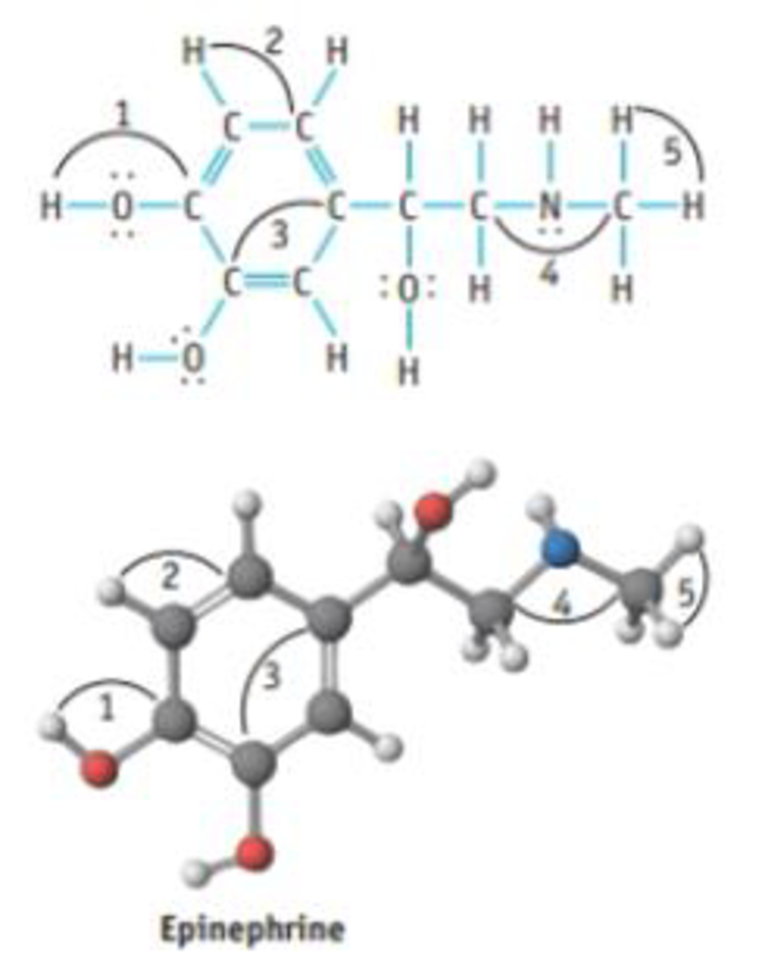# The molecule pictured below is epinephrine, a compound used as a bronchodilator and antiglaucoma agent. (a) Give a value for each of the indicated bond angles. (b) What are the most polar bonds in the molecule?### Chemistry & Chemical Reactivity

9th Edition
John C. Kotz + 3 others
Publisher: Cengage Learning
ISBN: 9781133949640

#### Solutions

Chapter
Section### Chemistry & Chemical Reactivity

9th Edition
John C. Kotz + 3 others
Publisher: Cengage Learning
ISBN: 9781133949640
Chapter 8, Problem 88GQ
Textbook Problem
83 views

## The molecule pictured below is epinephrine, a compound used as a bronchodilator and antiglaucoma agent.(a) Give a value for each of the indicated bond angles. (b) What are the most polar bonds in the molecule?

(a)

Interpretation Introduction

Interpretation: The bond angle in the given compound should be determined.

Concept Introduction:

In VSEPR, the geometry of the molecule is explained based on minimizing electrostatic repulsion between the molecules’ valence electrons around a central atom

Bond angle is the angle between two bonds of a molecule and it is determined based on the electron-domain geometry.

[Bond angles: tetrahedral = 109.5o, trigonal planar = 120o, T-shape = 90o]

Electron geometry is the shape of a molecule predicted by considering both bond electron pairs and lone pair of electrons.

Molecular geometry is the shape of a molecule predicted by considering only bond pair of electrons.

Bond angle: It is defined as the angle between the orbital contacting bonding electron pair around the central atom in a molecule ion. Bond angle is expressed in degree.

### Explanation of Solution

The compound is given below:

In bond angle 1, the geometry of central oxygen atom is bent due to the presence of two electron pairs on it. Hence, the bond angle of COH is 109.5o.

In bond angle 2 and 3, the geometry of central carbon atom is trigonal planar to minimize the electrostatic repulsion between the atoms. Hence, the bond angle of HCC and C=CC is 120o

(b)

Interpretation Introduction

Interpretation: The most polar bonds in the given compound should be determined.

Concept Introduction:

Polarity: It is a well separation of electric charge leading to a molecule or chemical compounds having an electrical dipole moment. Generally the polar molecules must contain polar bonds due to a different in electronegative between the bonded atoms.

### Still sussing out bartleby?

Check out a sample textbook solution.

See a sample solution

#### The Solution to Your Study Problems

Bartleby provides explanations to thousands of textbook problems written by our experts, many with advanced degrees!

Get Started

Find more solutions based on key concepts
_______ is/are removed from a new mRNA a. Introns b. Exons c. A poly-A tail d. Amino acids

Biology: The Unity and Diversity of Life (MindTap Course List)

The thermic effect of food plays a major role in energy expenditure, T F

Nutrition: Concepts and Controversies - Standalone book (MindTap Course List)

Which planktonic primary producers can succeed at the greatest depths?

Oceanography: An Invitation To Marine Science, Loose-leaf Versin

A person going for a walk follows the path shown in Figure P3.35. The total trip consists of four straight-line...

Physics for Scientists and Engineers, Technology Update (No access codes included)